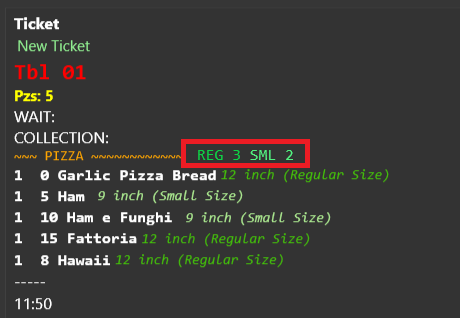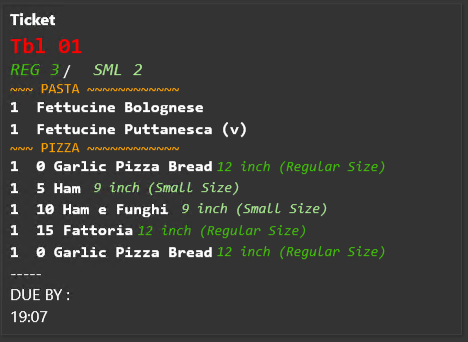# Setting up a Portion Counter in KDS

Reposting as AD for support request.

I need some help in adding a portion counter in the KDS, here is a mockup:I have tried to add this line to the current template:

REG: {REPORT ORDER DETAILS:O.Quantity.Sum.desc:(ODI=True) and (MG=PIZZA) and O.PortionName=“12 inch (Regular Size)” } / SML: {REPORT ORDER DETAILS:O.Quantity.Sum.desc:(ODI=True) and (MG=PIZZA) and O.PortionName=“9 inch (Small Size)” }

and it shows the total pizza for the work period, I need to restrict it to the current order but I’m out of my depth and need some professional support to get it done.

Thanks
Claudio

In case someone else looks for this here is a solution that worked for me:

>REG [=TN('{TICKET ORDER COUNT EXP:(ODI=True) and O.PortionName="12 inch (Regular Size)"}') / SML [=TN('{TICKET ORDER COUNT EXP:(ODI=True) and O.PortionName="9 inch (Small Size)"}')]

Here how it looksHere the full KDS print Template i’m using:

[LAYOUT]
{TICKET TYPE}
<size 22>{ENTITY NAME:Table}
<color #43c109><size 18>REG [=TN(‘{TICKET ORDER COUNT EXP:(ODI=True) and O.PortionName=“12 inch (Regular Size)”}’)] / <color #Adec91><size 18> SML [=TN(‘{TICKET ORDER COUNT EXP:(ODI=True) and O.PortionName=“9 inch (Small Size)”}’)]
{ORDERS}
DUE BY :[=FD(ADS(‘{TICKET DATE:HH:mm}’,+{TICKET TAG:Pickup Minutes}*60),‘hh:mm tt’)]
{TIME}

[ORDERS GROUP|PRODUCT GROUP]
<size 14>[=‘~~~ {GROUP KEY} ~~~~~~~~~~~~’.substr(0,40)]

[ORDERS]
<size 16>[=(‘{QUANTITY}’+’ ‘).substr(0,2)] {PRODUCT NAME} [=’{PORTION}‘==‘12 inch (Regular Size)’ ? ‘<color #43c109><size 14>{PORTION}’ : ‘’][=’{PORTION}'==‘9 inch (Small Size)’ ? ‘ <color #Adec91><size 14>{PORTION}’ : ‘’]
{SORTED ORDER TAGS}

[ORDERS:PRODUCT TAG:White Pizza=Yes]
<size 16>[=(‘{QUANTITY}’+’ ‘).substr(0,2)] {PRODUCT NAME}[=’{PORTION}‘==‘12 inch (Regular Size)’ ? ‘<color #43c109><size 14>{PORTION}’ : ‘’][=’{PORTION}'==‘9 inch (Small Size)’ ? ‘ <color #Adec91><size 14>{PORTION}’ : ‘’]
{SORTED ORDER TAGS}

[SORTED ORDER TAGS:Calzone Option]
<size 20>[=(‘{ORDER TAG QUANTITY}’>1 ? {ORDER TAG QUANTITY} : ’ ')] {ORDER TAG NAME}

{ORDER TAGS}

[ORDER TAGS]

• {ORDER TAG QUANTITY} {ORDER TAG NAME}

[TICKET TAGS]
{TICKET TAG NAME}
<size 20>Table:{ENTITY NAME:Table}